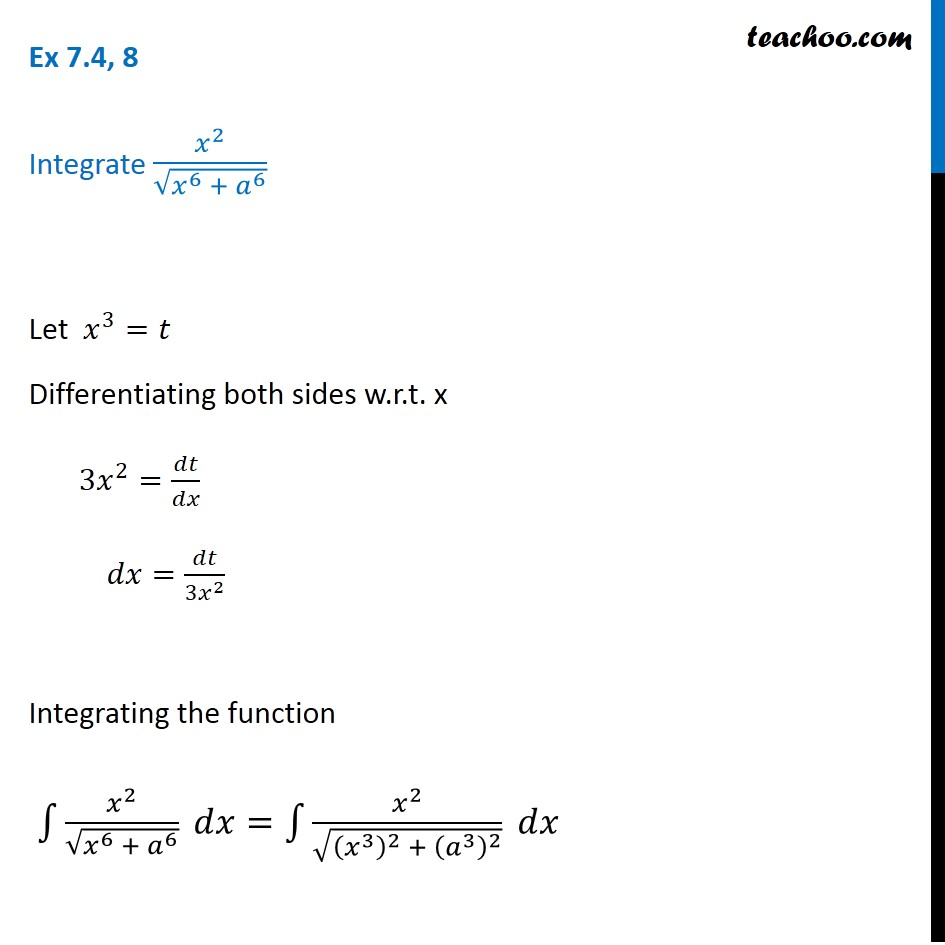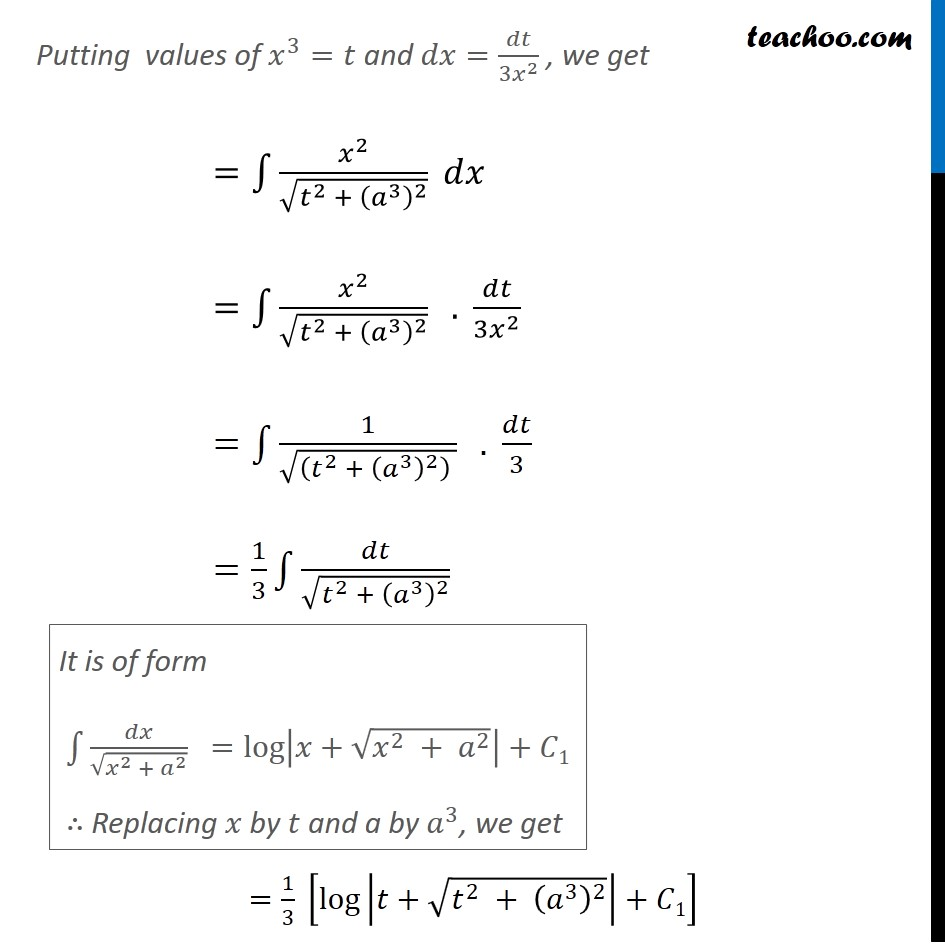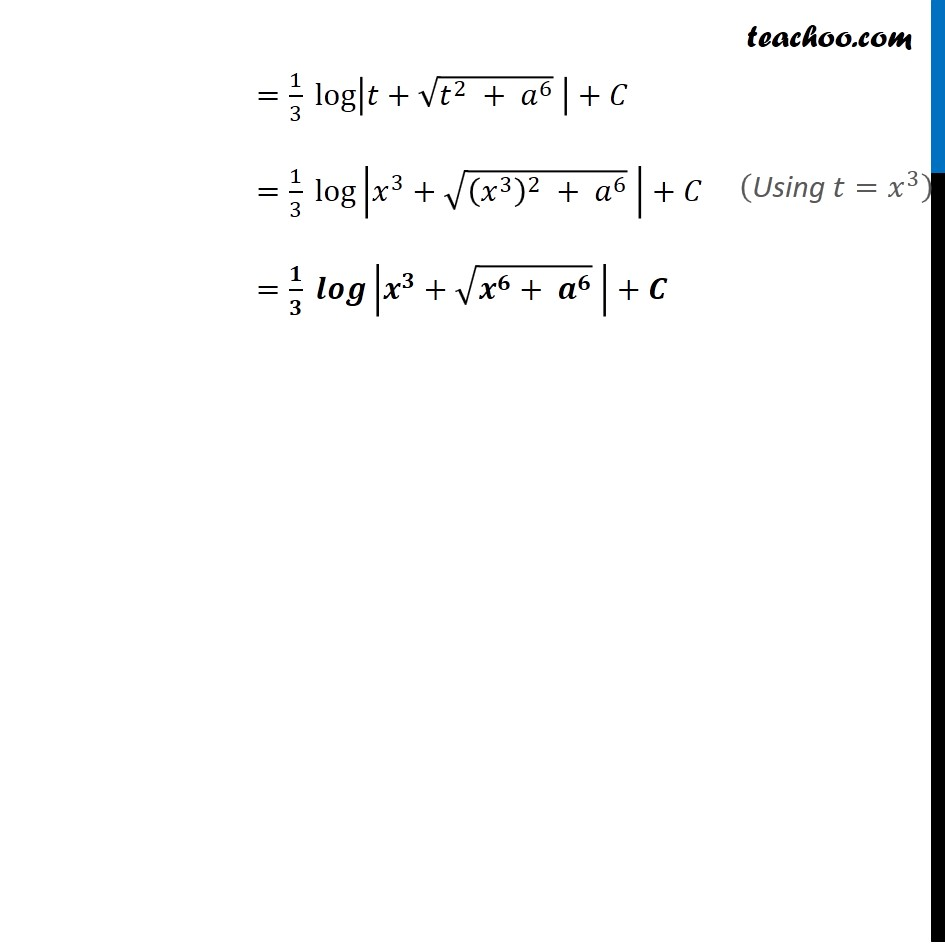Ex 7.4

Chapter 7 Class 12 Integrals
Serial order wiseLearn in your speed, with individual attention - Teachoo Maths 1-on-1 Class

### Transcript

Ex 7.4, 8 Integrate 𝑥^2/√(𝑥^6 + 𝑎^6 ) Let 𝑥^3=𝑡 Differentiating both sides w.r.t. x 3𝑥^2=𝑑𝑡/𝑑𝑥 𝑑𝑥=𝑑𝑡/(3𝑥^2 ) Integrating the function ∫1▒𝑥^2/√(𝑥^6 + 𝑎^6 ) 𝑑𝑥=∫1▒𝑥^2/√((𝑥^3 )^2 + (𝑎^3 )^2 ) 𝑑𝑥 Putting values of 𝑥^3=𝑡 and 𝑑𝑥=𝑑𝑡/(3𝑥^2 ) , we get =∫1▒𝑥^2/√(𝑡^2 + (𝑎^3 )^2 ) 𝑑𝑥 =∫1▒𝑥^2/√(𝑡^2 + (𝑎^3 )^2 ) . 𝑑𝑡/(3𝑥^2 ) =∫1▒1/√((𝑡^2 + (𝑎^3 )^2 ) ) . 𝑑𝑡/3 =1/3 ∫1▒𝑑𝑡/√(𝑡^2 + (𝑎^3 )^2 ) =1/3 [log⁡|𝑡+√(𝑡^2 + (𝑎^3 )^2 )|+𝐶1] It is of form ∫1▒𝑑𝑥/√(𝑥^2 + 𝑎^2 ) =log⁡|𝑥+√(𝑥^2 + 𝑎^2 )|+𝐶1 ∴ Replacing 𝑥 by 𝑡 and a by 𝑎^3, we get =1/3 log⁡|𝑡+√(𝑡^2 + 𝑎^6 ) |+𝐶 =1/3 log⁡|𝑥^3+√((𝑥^3 )^2 + 𝑎^6 ) |+𝐶 =𝟏/𝟑 𝒍𝒐𝒈⁡|𝒙^𝟑+√(𝒙^𝟔+ 𝒂^𝟔 ) |+𝑪 ("Using" 𝑡=𝑥^3 )